# Section 14.4. Performing Table Calculations

### 14.4. Performing Table Calculations

Excel tables have several nifty features that help you out when you're performing calculations on the data inside a table.

One example's the way that automatic expansion works when you're adding new columns that contain calculations. For example, say you've got a table with three columns : Product ID, Model Name , and Price. If you want to add a new column that tracks the discounted price of each product (say, 90 percent of the regular price), then a table's a great timesaver. Once you've created the new discount price column for one row (see Figure 14-21), Excel fills the same calculation into every other row (Figure 14-22). You wind up with a table that shows the discounted price of every product. No copying and pasting required.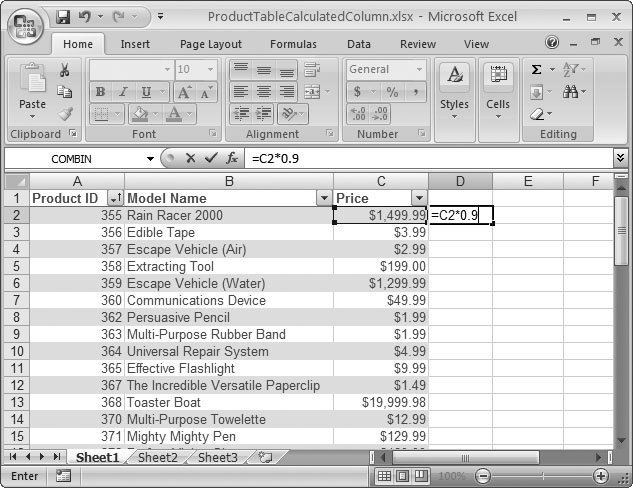Figure 14-21. This worksheet shows a new column with a formula that's in the process of being entered. See Figure 14-22 for what happens next .

Once you've added a new column with a calculation, the only thing left to do is to give it a good name. Click the column header cell (which will say something like Column1), and then type in something more relevant (like Discount Price).

#### 14.4.1. Dynamic Calculations

One of Excel tables' nicest features is the way they handle calculations. You can build formulas that use the data in a table, and Excel adjusts them automatically as the table grows or shrinks.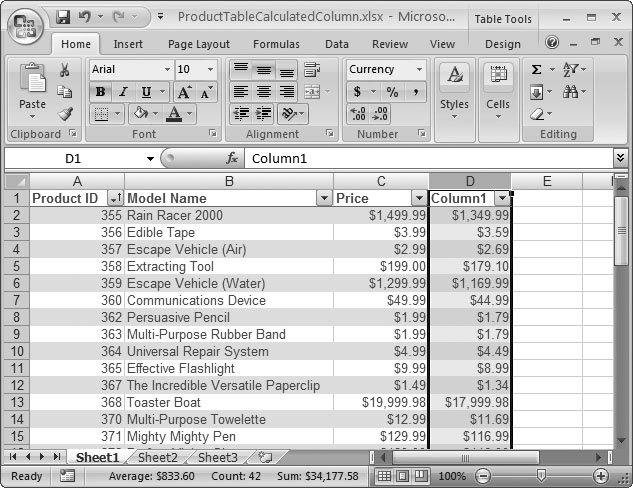Figure 14-22. Once you finish the formula shown in Figure 14-21, and then hit Enter, Excel expands the table to include the new column, and then fills the formula down to include every row. And if you scroll to the bottom of the table and start adding a new row, Excel is intelligent enough to automatically copy this new formula into the new row. In other words, once you add a formula to a column, that formula is sticky (Section 14.1.3).

For example, imagine creating a table that stretches from row 1 to row 5. The first row contains the column headers, followed by four product rows. You create the following formula to add the total price of all the items:

` =SUM(C2:C5) `

If you now add a new item (that is, a new row) to the table, Excel automatically updates your formula so it includes this new item:

` =SUM(C2:C6) `

The same magic happens if your table shrinks because you've deleted an item. You just need to make sure that your range includes the whole column when you first create the formula. For example, consider the following formula, which omits the first item in the table:

` =SUM(C3:C5) `

If you expand the table now, Excel doesn't modify the formula. Fortunately, the program's got your back: It'll remind you if you create a formula that includes only a portion of your table by showing a green triangle in the corner of the cell, which is Excel code for "Is this really what you want to do?" When you move to the cell, an exclamation mark icon appears. Click it, and you get a short explanation and a menu of error-fixing options, as shown in Figure 14-23.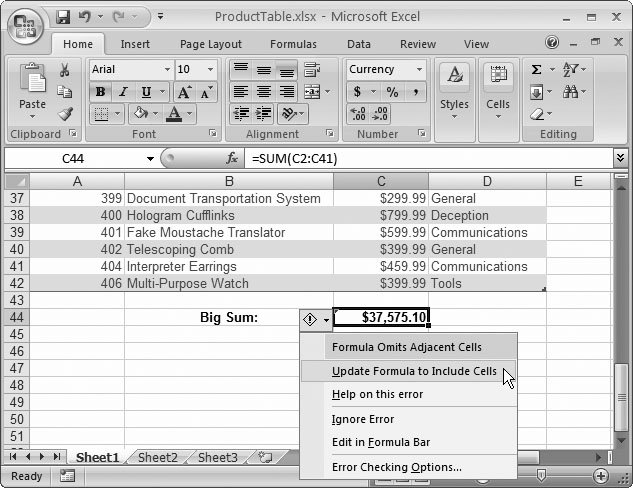Figure 14-23. Excel warns you if you create a function that leaves out part of the information in a table. For example, the formula in C44 mistakenly left out cell C42. Excel offers to remedy the problemjust choose Update Formula to Include Cells .

Filtering settings don't affect your formulas. If you filter the table so that only some products are visible, the SUM( ) function still produces the same result, which is the total price of all products. If you don't want this behavior, you can use the total row (Section 14.4.4) or Excel's SUBTOTAL( ) function (Section 14.4.5).

#### 14.4.2. Column Names

So far, the table calculation examples you've seen have ignored a super- convenient feature called column names . You've already learned how Excel lets you create your own named ranges to refer to frequently used parts of your worksheet. (Section 13.2 has the full story.) But even if you don't create any named ranges of your own, you'll find that Excel equips every table with some built-in names.

First of all, Excel creates column names that you can use when you write formulas inside your table. For example, look back at Figure 14-21, which uses this formula to discount the current price:

` =C2*0.9 `

When Excel copies this formula down the table, it adjusts the formula automatically using the familiar magic of relative references (Section 8.3.1), so that each discounted priced refers to the product price in the same row. For example, in row 10 (technically, the ninth row of data), the formula becomes:

` =C10*0.9 `

But there's a shortcut. Instead of referring to the specific cell, you can use the name of the column (which is whatever text you've placed in the column header) inside square brackets. That shortcut means you can write the formula like this:

` =[Price]*0.9 `

The [Price] name automatically refers to the value of the Price column in the current row, no matter where in the table you stick it.

Column names are not only major timesavers, they also make your worksheets much easier to understand. After all, who could mistake the following formula?

` =[Price]*[Tax Rate]+[Shipping Charge] `

Note: If you use Excel's point-and-click formula creation, Excel uses the column names rather than the cell reference.

#### 14.4.3. Table Names

You can't use column names in formulas that are in other parts of your worksheet. After all, Excel would have no idea what row you're trying to use. However, Excel gives you another shortcut. You can refer to your entire table anywhere on your worksheet by name .

This raises one excellent questionnamely, how does Excel decide what name your table should have? When you first create a table, Excel picks a rather unexciting name like Table1, Table2, and so on. To change this name, click anywhere inside your table, and then edit the text in the Table Tools Design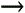PropertiesTable Name box. For example, ProductList makes a good name.

On its own, your table name refers to the entire range of cells that contains the data for your table. That means it includes the entire table, minus the column headers. You may want to use these cells with a lookup function like VLOOKUP( ), as described in Section 12.1.1. For example, if you want to get the price (from the third column) of the product named Persuasive Pencil, you can use this easy-to-read formula:

` =VLOOKUP("Persuasive Pencil", ProductList, 3, FALSE) `

Excel includes another treat that lets you dig deeper into your table. You can use the table name in conjunction with a column name to get the range of cells that holds the data for just one column. Here's an example:

` =SUM(ProductList[Price]) `

This gets the ProductList table, pulls out the cells in the Price column, and then passes them to the familiar SUM( ) function, which generates a total. You can quickly and efficiently get a hold of parts of a table. Figure 14-24 shows you how Excel helps you create formulas like these with its Formula AutoComplete feature.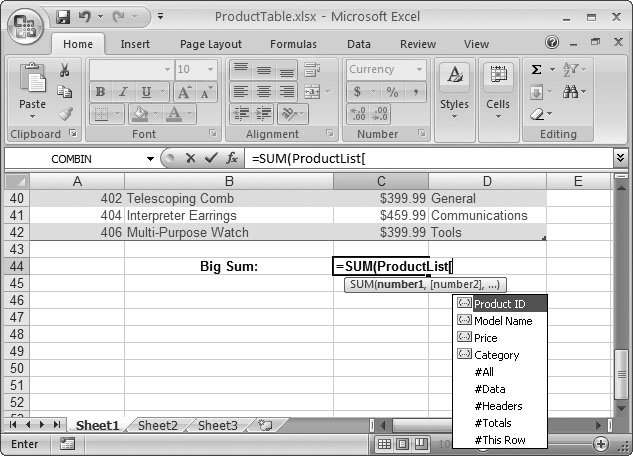Figure 14-24. Once you type a table name followed by an open bracket , Excel shows a list of columns in that table. You can select one using the up and down arrow keys, and then insert it into your formula by pressing Tab.

#### 14.4.4. The Total Row

Excel tables make it easy to calculate totals, averages, standard deviations, and other common formulas by using a dedicated summary row. To show this row, just select Table Tools DesignTable Style OptionsTotal Row. Excel adds an extra row at the bottom of the table.

When the total row first appears, it shows only one piece of information: the number of records currently displayed. If you want to show some other type of information, choose a column, and then click the total row cell at the bottom of that column. A drop-down list appears with preset options. Choose one, and the total row displays the calculation in that cell, as shown in Figure 14-25.

Of course, you can create your own formulas to show the same information as the total rows do. However, the total row requires no work. It also uses only the rows that are currently visible, ignoring all filtered rows. You may or may not want this behavior. (It's great if you want to calculate totals for a small subset of filtered data, but it's not so good if you want to create grand totals that include everything.) If you don't want this behavior, you can write your own summary formula using functions like SUM( ) (Section 9.2), COUNT( ) (Section 9.2.2), and AVERAGE( ) (Section 9.2.5).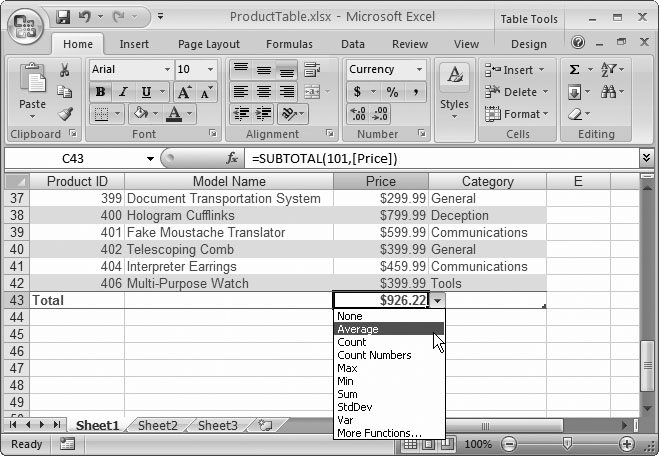Figure 14-25. The Total row lets you perform common calculations with a single mouse click. In this example, the Total row displays the average price out of the three records that matched the custom filter condition.

#### 14.4.5. The SUBTOTAL() Function

The total row conveniently works only with the currently visible rows in a table. To see this phenomenon in action, simply click one of the cells in the total row. If you look in the formula bar, you see that these cells use the SUBTOTAL() function to perform their calculations. That's because the SUBTOTAL() function is the only Excel function that takes table filtering into account.

The SUBTOTAL() function is the perfect solution for all the calculations in the total row, including sums, averages, counts, and more. The trick is that the first argument of the SUBTOTAL() function is a numeric code that tells Excel what type of calculation it should perform, while the second argument is the range of cells for the entire table column, from the first row to the last.

` SUBTOTAL(function_code, column_range) `

Table 14-1 lists all the function codes you can use with SUBTOTAL(). Note that each calculation type actually has two function codes associated with it. Function codes above 100 ignore hidden rows (rows that you've hidden using the HomeCellsFormatHide & UnhideHide Rows command). These function codes are the ones the total row uses. Function codes under 100 don't ignore hidden rows, but they still ignore rows you've filtered out. Figure 14-26 shows the SUBTOTAL() function in action.

##### Table 14-1. Function Codes for SUBTOTAL()

Function Used for Calculation

Code That Ignores Hidden Rows

Code That Includes Hidden Rows

AVERAGE()

101

1

COUNT()

102

2

COUNTA()

103

3

MAX()

104

4

MIN()

105

5

PRODUCT()

106

6

STDEV()

107

7

STDEVP()

108

8

SUM()

109

9

VAR()

110

10

VARP()

111

11

In Figure 14-26, compare the results of the SUBTOTAL() functions in cells C2 to C6 to the formulas in cells D2 to D6, which just use the regular functions, like AVERAGE(), SUM(), MAX(), MIN(), and so on. In this figure, the formulas show the totals after filtering's limited the table to items in the Communications category.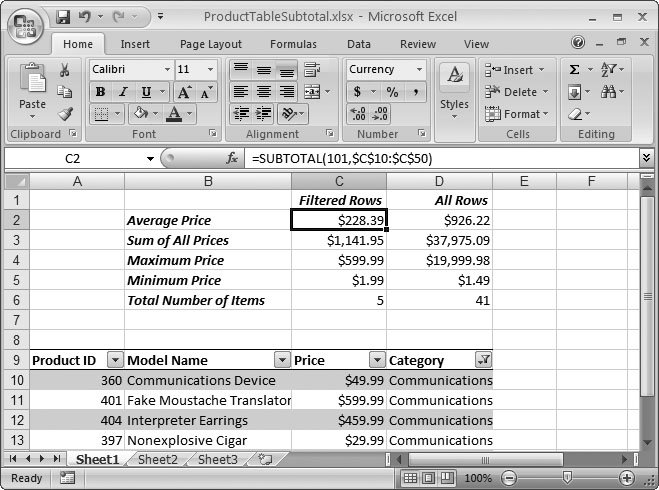Figure 14-26. This worksheet puts the SUBTOTAL() function to work calculating averages, sums, and more. The formulas that use the SUBTOTAL() function are in cells C2 to C6, while the formulas that use the closest ordinary Excel function (and don't take filtering into account) are in cells D2 to D6.

#### 14.4.6. The Database Functions

Excel also includes functions exclusively for use with long tables. These are the database functions , a set of 11 functions that let you analyze groups of data.

The database functions are very similar to the basic statistical functions like SUM(), AVERAGE(), and COUNT(). In fact, the database functions have the exact same names, but with an initial letter Dso you find a DSUM(), DAVERAGE(), DCOUNT(), and so on. The database functions differ from their non-database counterparts in that they can selectively filter out rows. In other words, when you use DSUM(), you can specify a set of criteria that a record must match in order to be included in the sum. (The filtering that you've applied with the AutoFilter feature makes no difference to the database functions. They don't ignore hidden rows.)

Table 14-2 lists the database functions, along with comparable statistical function.

##### Table 14-2. Database Functions

Function

Similar To

Description

DAVERAGE()

AVERAGE()

Calculates the average in rows that meet the specified criteria.

DCOUNT()

COUNT()

Counts the number of rows that meet the specified criteria.

DCOUNTA()

COUNTA()

Calculates the number of non-blank values in rows that meet the specified criteria.

DGET()

No equivalent

Returns the value that meets the specified criteria. If more than one value matches, DGET() returns the #NUM ! error. If no records match, it returns the #VALUE ! error.

DMAX()

MAX()

Returns the maximum value in rows that meet the specified criteria.

DMIN()

MIN()

Returns the minimum value in rows that meet the specified criteria.

DPRODUCT()

PRODUCT()

Calculates the product produced by multiplying all values in rows that meet the specified criteria.

DSTDEV()

STDEV()

Calculates the standard deviation in rows that meet the specified criteria.

DSUM()

SUM()

Calculates the sum of values in rows that meet the specified criteria.

DVAR()

VAR()

Estimates the variance of a sample population in the rows that meet the specified criteria.

DVARP()

VARP()

Estimates the variance of an entire population in the rows that meet the specified criteria.

DGET() is the only function without a statistical counterpart . DGET() works a little like the VLOOKUP() and HLOOKUP() functions (Section 12.1), and it returns a single value in a row that meets the specified criteria.

Each database function uses the exact same three parameters:

` DFUNCTION(table_range, field, criteria) `

• The table_range is the range that the function uses. The table range should include the entire table, including the column you want to use for your calculation and the columns to use for your criteria. The table range must include the column headers, because that's how the database functions identify each column and match it up with the criteria. That means you can't use the automatically generated table names you learned about earlier (Section 14.4.3).

• The field is the name of the column you want to use for the calculation. For example, if you're using DSUM(), the field is the numeric column you want to total. Excel scans the column headers until it finds the column that has the same name.

• The criteria is a range of cells that specifies all the conditions that rows must meet to be included in the calculation. This range can be as large as you want, and you're free to define conditions for multiple columns, or multiple conditions that apply to the same column. If the range contains only empty cells, the database function operates on all the items in the table.

To use a database function successfully, you need to create a suitable range of cells that you can use for criteria. Excel expects this range of cells to be in a strict arrangement. Here are some rules you'll need to follow:

• Each condition needs two cells. One cell specifies the name of the field, and the other specifies the filter condition. For example, you can enter "Category" for the field name and "Tools" for the filter condition.

• The cell with the filter condition must be directly under the cell with the field name. If you put them side by side, the database function just gives you an error.

• You can add as many conditions as you want. You can even use multiple criteria that impose different conditions on the same columns. However, you must place them in columns next to each other. You can't stack them on top of one another.

Filter conditions follow the same rules that you used for search criteria in the data form window. Thus, you can use comparison operators like less than (<) and greater than (>) to create conditions like < 500 (all prices under \$500) or <> Travel (all products not in the Travel category). If you don't specify a comparison operator, Excel acts as though you've chosen the equal sign. In addition, it allows partial matches with text criteria. For example, the category criteria T will match both Travel and Tools.

You have total freedom to decide where on the worksheet to place your filter cells. Figure 14-27 shows an example that puts the filter cells at the top of the worksheet. To find the average price of all the products that match these criteria, you'd use the following formula:

` =DAVERAGE(A9:D51, "Price", C1:E2) `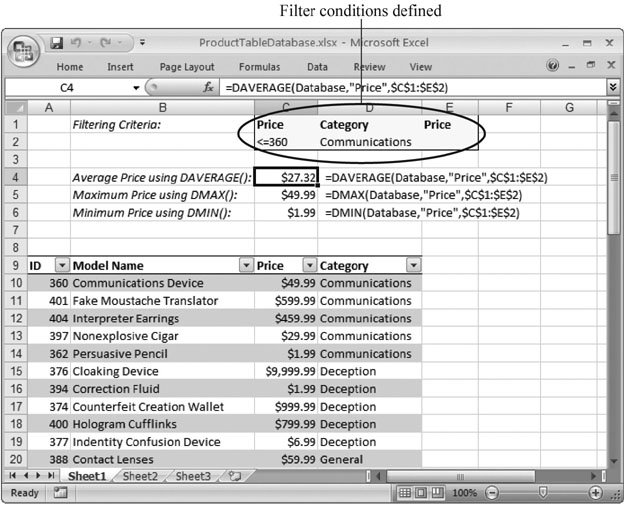Figure 14-27. In this example, you could use cell E2 to add another condition specifying a minimum price. However, because this cell is currently blank, Excel doesn't apply a price filter. Instead, it filters by matching a category and setting a maximum price.

The database functions are nice because they force you to define your criteria on the worksheet. If you want to change the criteria, you simply need to modify the condition cells, which makes the database functions a perfect solution for building dynamic reports .Excel 2007[c] The Missing Manual
ISBN: 596527594
EAN: N/A
Year: 2007
Pages: 173